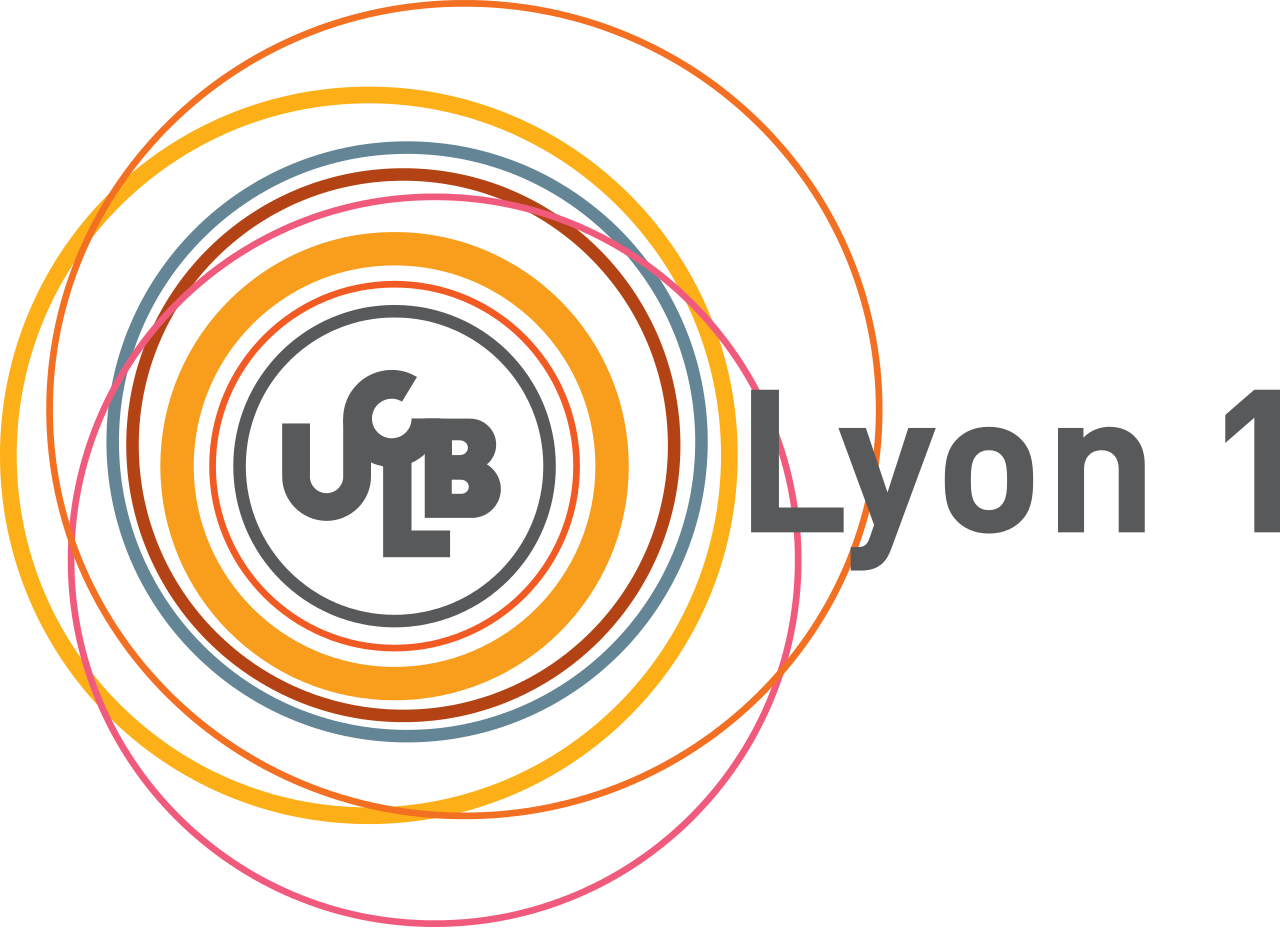# Scientific Focus###### AERES's visit

Laure Gonnord, 18 nov. 2014

## Research Topics

### Quantitative analysis of programs

• Numerical invariant generation for safety

• Formal methods for Software Engineering

• Reactive systems: synchronous design

• Compilation: scheduling, termination of programs

## Zoom : Termination of programs

### Why ?

• Reactive systems

• Proving full correction

• Because termination is related to scheduling

### The big picture

Given a program

//N>0
i = N;
while(i>0)
{
j = N;
while(j>0) j--;
i--;
}

Compute a model (or intermediate representation)

Prove termination of the model.

### From Hoare rules to ranking functions

• Hoare rule for total correctness:
• $$\frac{\{t=z \mbox{ and }{t\in D} \mbox{ and } P \mbox { and }B\}S\{P\mbox{ and }t<z\mbox{ and }t\in D\}}{\{\mbox{P}\} \mbox{while B do S done} \{\mbox{not B and P}\}}$$
• with $$(D,<)$$ well founded

• In our case:
• $$D=(\mathbb{N}^d,<_{lex})$$ and
• we look for $$\rho(k,-)$$ positive and strictly decreasing.
• we need (numerical) invariants $${\cal I}_k$$!

### Algorithm (Alias, Darte, Feautrier, Gonnord 2010) :

• From the program representation, we build a system of constraints:

• $$\rho$$ is positive : $$\rho(k,\vec{x})\geq 0$$ on $${\cal I}_{k}$$
• $$\rho$$ is decreasing : $$\vec{x'}-\vec{x^{}}\in \tau \Rightarrow \rho(dest,\vec{x'})-\rho(src,\vec{x^{}})<0$$
• We transform it into a Linear Programming instance

• We solve.

### Issues of the first implementation

Rank tool on compsys-tools.ens-lyon.fr

• applicability: only numerical programs
• abstraction of all non-numerical statements
• not enough!

• scalability:
• complexity is $$O(nbvars*nbcontrolpoints*dim)$$
• LP instances are too big
• using slicing is not enough

### Fighting against scalability : overview

• [WST14] (with F. Pereira, Brasil): realworld loops are simple !
• slice and pattern-match

• [Submitted to PLDI] (with D. Monniaux, Grenoble) : only a few assignments in a loop actually matter !
• Consider loops as single transitions and only heads of loops
• Compute incrementally the LP-problem.

## Conclusion & Future Work

• Improvement of the state of the art thanks to methods coming from:
• Polyhedral scheduling (Feautrier)
• Static analysis (Abstract interpretation, $$\ldots$$)
• Logic : SMT solvers, optimising SMT solvers

• Future work:
• scalability : consolidate Termite's implementation. (D. Monniaux, G. Radanne)
• applicability : look at rewriting techniques for termination of recursive functions, or complex data structures (C. Fuhs, Plume)